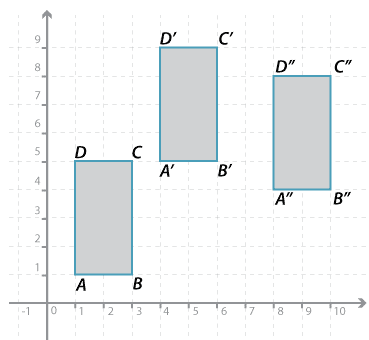#### Question 4Detailed description

1. Describe the translation that takes rectangle $$ABCD$$ to $$A^\prime B^\prime C^\prime D^\prime$$.
The translation is to the right and up.
2. Describe the translation that takes rectangle $$A^\prime B^\prime C^\prime D^\prime$$ to $$A^{\prime\prime} B^{\prime\prime} C^{\prime\prime} D^{\prime\prime}$$.
The translation is to the right and down.
3. Describe the translation that takes rectangle $$ABCD$$ to $$A^{\prime\prime} B^{\prime\prime} C^{\prime\prime} D^{\prime\prime}$$.
The translation is to the right and up.

Place answer in both checkboxes in the row before submitting.